### Updating equations for the prior site type probabilities

We first derive the updating equations for the prior site type probabilities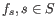. The probability that the site is of type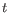given that we observe the nucleotides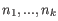in the reads at the site is: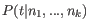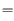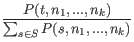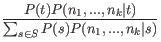(28.4)

Now, for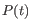we use our current value for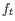, and if we further insert the expression for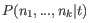(28.2) we get: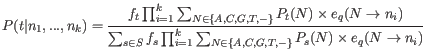(28.5)

We get the updating equation for the prior site type probabilities,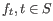, from equation 28.5: Let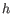index the sites in the alignment (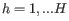). Given the current values for the set of site frequencies,, and the current values for the set of error probabilities, we obtain updated values for the site frequencies,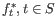, by summing the site type probabilities given the data (as given by equation 28.5) across all sites in the alignment: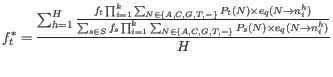(28.6)